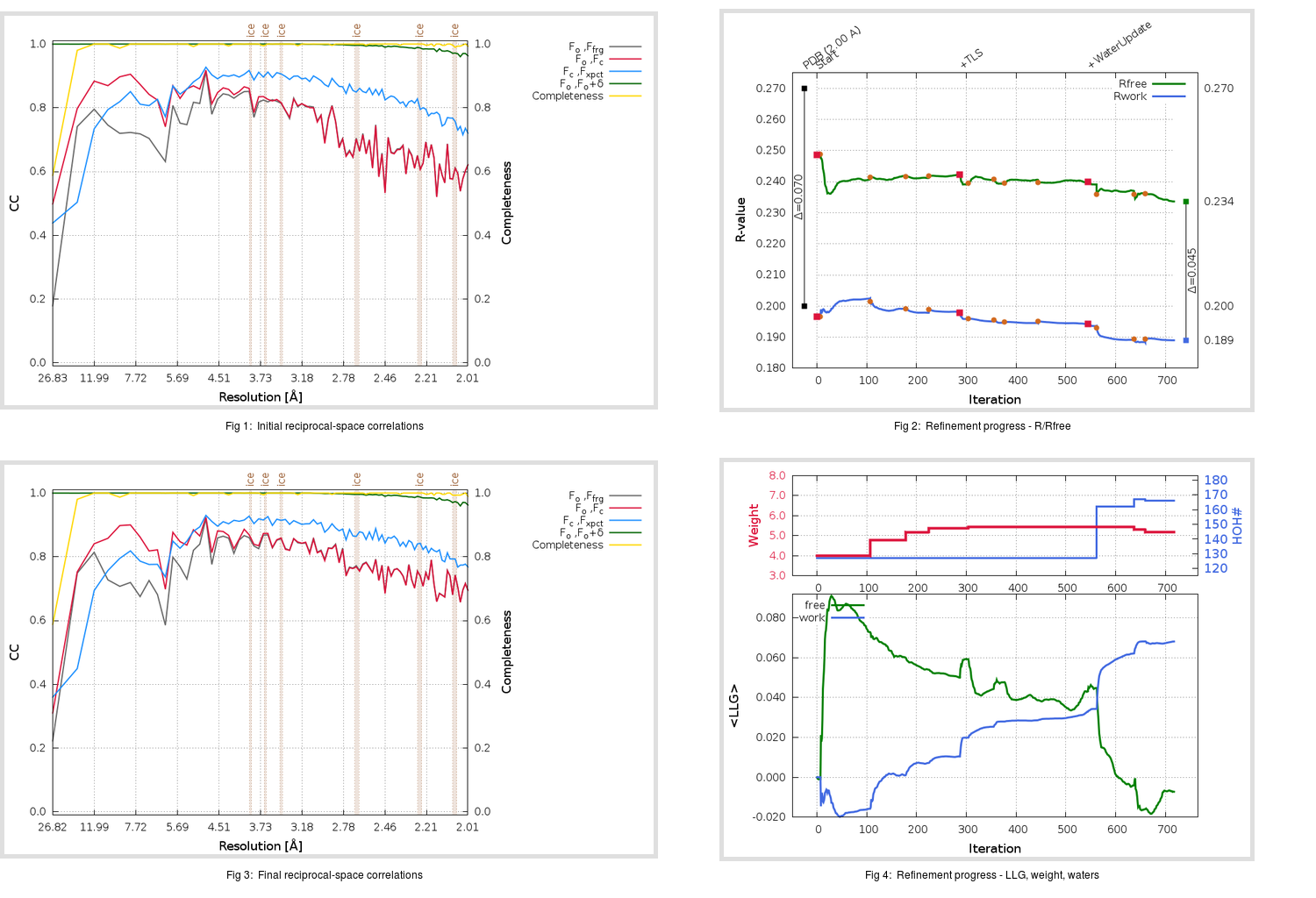Content:

```    Diffraction limits & principal axes of ellipsoid fitted to diffraction cut-off surface:
1.964         1.0000   0.0000   0.0000       a*
1.964         0.0000   1.0000   0.0000       b*
1.974         0.0000   0.0000   1.0000       c*
```

## Deposited

` `
 Date deposited Date data collection Resolution R, Rfree 20060427 20040825 2.00 0.1960 0.2700

Molprobity (CCP4 7.0 version) summary:

```Ramachandran outliers =   1.35 %
favored =  95.29 %
Rotamer outliers      =   8.88 %
C-beta deviations     =     7
Clashscore            =   9.95
RMS(bonds)            =   0.0220
RMS(angles)           =   2.03
MolProbity score      =   2.57
Resolution            =   2.00
R-work                =   0.1960
R-free                =   0.2700
```

```Number of waters      =   127

<B> (all atoms) =   24.35 ( sd =    5.35 ) for       2509 non-hydrogen atoms
<B>   (protein) =   23.93 ( sd =    4.84 ) for       2332 non-hydrogen atoms
<B>     (water) =   32.57 ( sd =    8.05 ) for        127 non-hydrogen atoms
<B>    (others) =   23.28 ( sd =    2.51 ) for         50 non-hydrogen atoms

B min/max       (all non-hydrogen atoms) =   10.38 /   48.56
B min/max   (protein non-hydrogen atoms) =   10.38 /   46.63
B min/max     (water non-hydrogen atoms) =   14.65 /   48.56
B min/max     (other non-hydrogen atoms) =   15.38 /   37.89
```

## BUSTER (re-)refinement

` `

Molprobity (CCP4 7.0 version) summary:

```Ramachandran outliers =   0.67 %
favored =  95.96 %
Rotamer outliers      =   6.95 %
C-beta deviations     =     1
Clashscore            =   2.54
RMS(bonds)            =   0.0116
RMS(angles)           =   1.64
MolProbity score      =   1.96
Resolution            =   2.00
R-work                =   0.1892
R-free                =   0.2339
```

```Number of waters      =   166

<B> (all atoms) =   27.68 ( sd =    7.82 ) for       2548 non-hydrogen atoms
<B>   (protein) =   26.97 ( sd =    7.23 ) for       2332 non-hydrogen atoms
<B>     (water) =   36.97 ( sd =   10.07 ) for        166 non-hydrogen atoms
<B>    (others) =   29.94 ( sd =    5.78 ) for         50 non-hydrogen atoms

B min/max       (all non-hydrogen atoms) =    8.13 /   69.82
B min/max   (protein non-hydrogen atoms) =   14.78 /   58.94
B min/max     (water non-hydrogen atoms) =   16.22 /   69.82
B min/max     (other non-hydrogen atoms) =    8.13 /   35.16
```

Refinement progression:Results:

` `
 File Remark 2GTB_aB_refine.01_03_refine.pdb.gz exact refinement commands are in header 2GTB_aB_refine.01_03_refine.mtz.gz including original deposited data and several re-refinement map coefficients 2GTB_aB_refine.01_03_BUSTER_model.cif.gz including any non-standard compound restraints 2GTB_aB_refine.01_03_BUSTER_refln.cif.gz